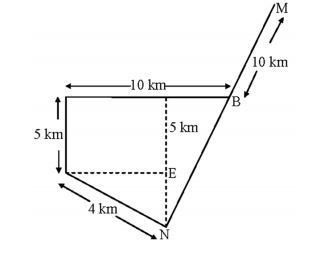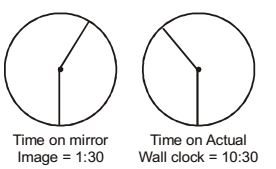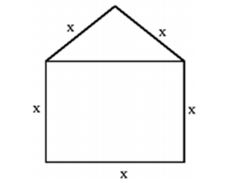## Numerical Ability

#### Numerical Ability

1. A couple has 2 children. The probability that both children are boys if the older one is a boy is

1. The probability of the second child being a boy is independent of the first child. Therefore, the required probability will be 1/2.

##### Correct Option: C

The probability of the second child being a boy is independent of the first child. Therefore, the required probability will be 1/2.

1. M and N start from the same location. M travels 10 km East and then 10 km North-East. N travels 5 km South and then 4 km South-East. What is the shortest distance (in km) between M and N at the end of their travel.

1. From the given data, the following diagram is possiblecos 45° = DE 4

DE = cos45° × 4 = 2.828 km
 sin 45° = EN 4

EN = sin 45° × 4 = 2.828 km
 sin 45° = EN 4

EN = sin 45° × 4 = 2.828 km
CN = NE + CE = 2.828 + 5 = 7.828 km
CB = AB – AC = 10 – 2.828 = 7.171 km
(NB)² = (NC)² + (BC)²
= (7.828)² + (7.171)²
∴ NB = √(7.828)² + (7.171)²
= 10.616 km
∴ NM = NB + BM = 10.616 + 10
= 20.61 km

##### Correct Option: C

From the given data, the following diagram is possiblecos 45° = DE 4

DE = cos45° × 4 = 2.828 km
 sin 45° = EN 4

EN = sin 45° × 4 = 2.828 km
 sin 45° = EN 4

EN = sin 45° × 4 = 2.828 km
CN = NE + CE = 2.828 + 5 = 7.828 km
CB = AB – AC = 10 – 2.828 = 7.171 km
(NB)² = (NC)² + (BC)²
= (7.828)² + (7.171)²
∴ NB = √(7.828)² + (7.171)²
= 10.616 km
∴ NM = NB + BM = 10.616 + 10
= 20.61 km

1. Two and a quarter hours back, when seen in a mirror, the reflection of a wall clock without number markings seemed to show 1:30. What is the actual current time shown by the clock?

1.So present time = 10:30 + 2:15
= 12:45

##### Correct Option: DSo present time = 10:30 + 2:15
= 12:45

1. A window is made up of a square portion and an equilateral triangle portion above it. The base of the triangular portion coincides with the upper side of the square. If the perimeter of the window is 6 m, the area of the window in m² is

1. The possible window will be as follows:Perimeter of window = 5x = 6m
x = 1.2 m
So area of window = Area of triangle + Area of square

 = √3 x² + x² 4

 = √3 (1.2)² + (1.2)² 4

= 2.06 m²

##### Correct Option: B

The possible window will be as follows:Perimeter of window = 5x = 6m
x = 1.2 m
So area of window = Area of triangle + Area of square

 = √3 x² + x² 4

 = √3 (1.2)² + (1.2)² 4

= 2.06 m²

1. The volume of a sphere of diameter 1 unit is than the volume of a cube of side 1 unit.

1. ‘Lesser than’ is apt because the sentence should be in comparative degree.

##### Correct Option: B

‘Lesser than’ is apt because the sentence should be in comparative degree.